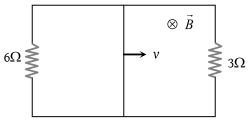# NEET Practice Questions• Subject:

...

• Chapter:

...

A rectangular loop with a sliding connector of length l = 1.0 m is situated in a uniform magnetic field B = 2T perpendicular to the plane of loop. Resistance of connector is r = 2Ω. Two resistance of 6Ω and 3Ω are connected as shown in figure. The external force required to keep the connector moving with a constant velocity v = 2m/s is(1) 6 N

(2) 4 N

(3) 2 N

(4) 1 N